Solution of Motion (Page No - 43) - Physics by Lakhmir Singh, Class 9

# Solution of Motion (Page No - 43) - Physics by Lakhmir Singh, Class 9 - Extra Documents & Tests for Class 9

Question 53:
The graph given alongside shows the positions of a body at different times. Calculate the speed of the body as it moves from :
(i) A to B,
(ii) B to C, and
(iii) C to D.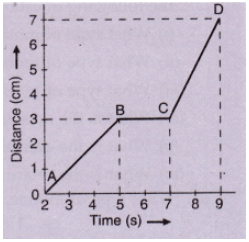Solution :
(i) The distance covered from A to B is( 3-0) =3 cm
Time taken to cover the distance from A to B =(5 -2) =3s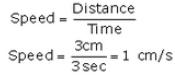(ii) The speed of the body as it moves from B to C is zero.
(iii) The distance covered from C to D is (7-3)=4 cm
Time taken to cover the distance from C to D = (9-7)=2s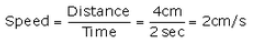Question 54:
What can you say about the motion of a body if:
(a) its displacement-time graph is a straight line ?
(b) its velocity-time graph is a straight line ?
Solution :
(a) The body has a uniform velocity if its displacement-time graph is a straight line.
(b) The body has a uniform acceleration if its velocity-time graph is a straight line.

Question 55:
A body with an initial velocity x moves with a uniform acceleration y. Plot its velocity-time graph.
Solution :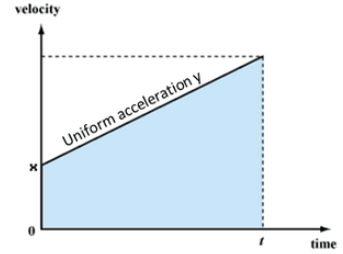Question 56:
Given alongside is the velocity-time graph for a moving body :
Find :
(i) Velocity of the body at point C.
(ii) Acceleration acting on the body between A and
(iii) Acceleration acting on the body between B and C.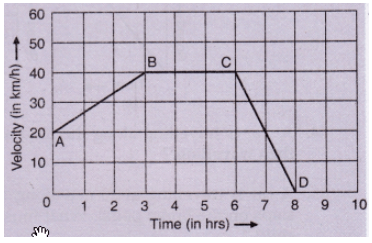Solution :
(i) BC represents uniform velocity. From graph, we see that the velocity of the body at point C = 40km/h
(ii) Acceleration between A and B = slope of line AB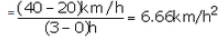(iii) BC represents uniform velocity, so acceleration acting on the body between B and C is zero.

Question 57:
A body is moving uniformly in a straight line with a velocity of 5 m/s. Find graphically the distance covered by it in 5 seconds.
Solution :
Distance travelled = Area of rectangle OABC
= OA x OC
= 5 x 5 =25 m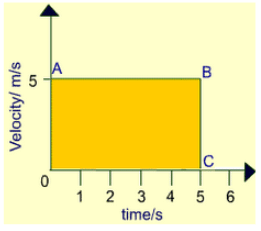Question 58:
The speed-time graph of an ascending passenger lift is given alongside.
What is the acceleration of the lift:
(i) during the first two seconds ?
(ii) between second and tenth second ?
(iii) during the last two seconds ?

Solution:

(i) Acceleration during first two seconds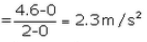(ii) Acceleration between second and tenth second is zero, since the velocity is constant during this time.

(iii) Acceleration during last two seconds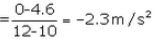Question 59:
A car is moving on a straight road with uniform acceleration. The speed of the car varies with time as follows :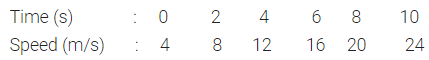Draw the speed-time graph by choosing a convenient scale. From this graph :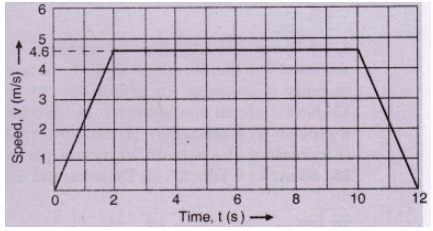(i) Calculate the acceleration of the car.
(ii) Calculate the distance travelled by the car in 10 seconds.
Solution :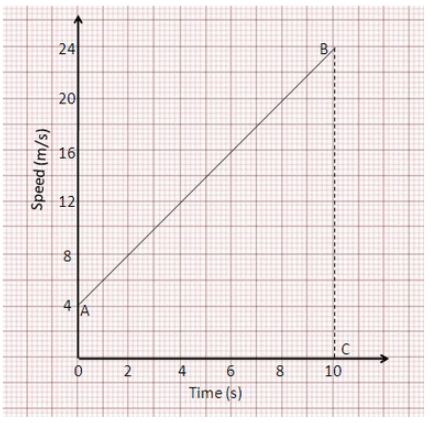(i) Acceleration of the car = slope of line AB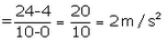(ii) Distance travelled by the car in 10 s = area of trapezium OABC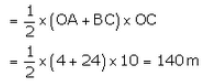Question 60:
The graph given alongside shows how the speed of a car changes with time: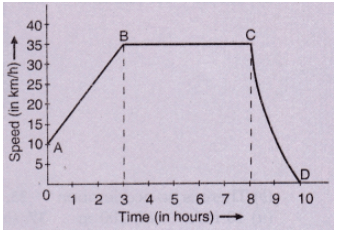(i) What is the initial speed of the car ?
(ii) What is the maximum speed attained by the car ?
(iii) Which part of the graph shows zero acceleration ?
(iv) Which part of the graph shows varying retardation ?
(v) Find the distance travelled in first 8 hours.
Solution :
(i) Initial speed of the car=10km/h
(ii) Maximum speed attained by the car= 35km/h
(iii) BC represents zero acceleration.
(iv) CD represents varying retardation.
(v)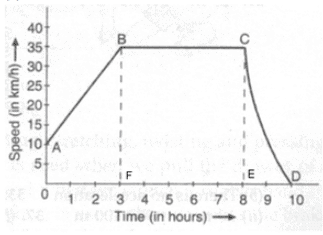Distance travelled in first 8 hrs:

s= Area of trapezium OABF + Area of rectangle BCEF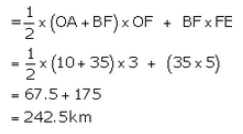Question 61:
Three speed-time graphs are given below :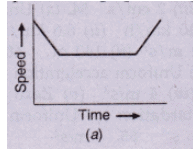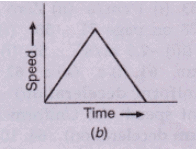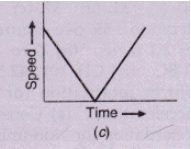Which graph represents the case of:
(i) a cricket ball thrown vertically upwards and returning to the hands of the thrower ?
(ii) a trolley decelerating to a constant speed and then accelerating uniformly ?
Solution :
(i) Graph (c): The speedof the ball goes on decreasing uniformly as it moves upward, reaches zero at the highest point, and then increases uniformly as it moves downward.
(ii) Grap(a): The speed of the trolley decreases uniformly, then it moves at a constant speed, and then the speed increases uniformly.

The document Solution of Motion (Page No - 43) - Physics by Lakhmir Singh, Class 9 | Extra Documents & Tests for Class 9 is a part of the Class 9 Course Extra Documents & Tests for Class 9.
All you need of Class 9 at this link: Class 9

## Extra Documents & Tests for Class 9

1 videos|228 docs|21 tests

## FAQs on Solution of Motion (Page No - 43) - Physics by Lakhmir Singh, Class 9 - Extra Documents & Tests for Class 9

 1. What is motion?Ans. Motion refers to the change in position of an object with respect to its surroundings over time. It can be described in terms of its speed, direction, and acceleration.
 2. What are the different types of motion?Ans. There are three main types of motion: 1. Translational motion: When an object moves along a straight line, it is called translational motion. 2. Rotational motion: When an object spins or rotates around an axis, it is called rotational motion. 3. Vibrational motion: When an object moves back and forth around a fixed position, it is called vibrational motion.
 3. What is the difference between speed and velocity?Ans. Speed refers to how fast an object is moving, while velocity refers to the speed of an object in a specific direction. Velocity takes into account the object's displacement, which is the change in its position with respect to time.
 4. What is the formula to calculate speed?Ans. The formula to calculate speed is: Speed = Distance/Time. Here, distance is the total path traveled by an object, and time is the duration taken to cover that distance.
 5. What is acceleration?Ans. Acceleration is the rate at which an object changes its velocity. It can be positive (when the object is speeding up) or negative (when the object is slowing down). The formula to calculate acceleration is: Acceleration = Change in velocity/Time taken.

## Extra Documents & Tests for Class 9

1 videos|228 docs|21 testsExplore Courses for Class 9 examSignup to see your scores go up within 7 days! Learn & Practice with 1000+ FREE Notes, Videos & Tests.
10M+ students study on EduRev
Track your progress, build streaks, highlight & save important lessons and more!
Related Searches

,

,

,

,

,

,

,

,

,

,

,

,

,

,

,

,

,

,

,

,

,

,

,

,

;